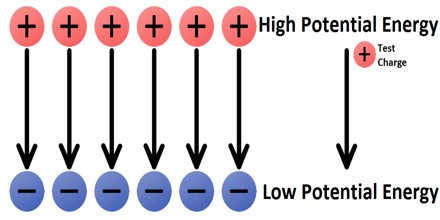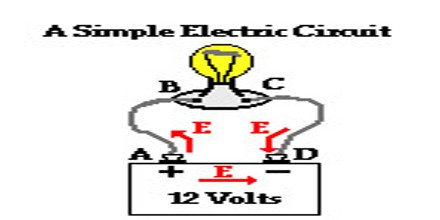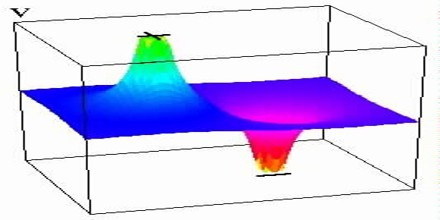Electric Potential

Definition

Electric Potential is the ‘push’ of electricity through a circuit. It’s easy to confuse electric potential with electric current, so it helps to think of electric current as the water in our shower and electric potential as the water pressure. Like water pressure, varying voltage can increase or decrease the flow of electricity.Electric Potential is a scalar quantity denoted by V, equal to the electric potential energy of any charged particle at any location (measured in joules) divided by the charge of that particle (measured in coulombs). By dividing out the charge on the particle a remainder is obtained that is a property of the electric field itself. This value can be calculated in either a static (time-invariant) or a dynamic (varying with time) electric field at a specific time in units of joules per coulomb, or volts (V). The electric potential at infinity is assumed to be zero.

The concept of electric potential is useful in understanding electrical phenomena; only differences in potential energy are measurable. If an electric field is defined as the force per unit charge, then by analogy an electric potential can be thought of as the potential energy per unit charge. Therefore, the work done in moving a unit charge from one point to another (e.g., within an electric circuit) is equal to the difference in potential energies at each point. In the International System of Units (SI), electric potential is expressed in units of joules per coulomb (i.e., volts), and differences in potential energy are measured with a voltmeter.Electric Potential in Circuits

Charge moving through the wires of the circuit will encounter changes in electric potential as it traverses the circuit. Within the electrochemical cells of the battery, there is an electric field established between the two terminals, directed from the positive terminal towards the negative terminal. As such, the movement of a positive test charge through the cells from the negative terminal to the positive terminal would require work, thus increasing the potential energy of every Coulomb of charge that moves along this path. This corresponds to a movement of positive charge against the electric field. It is for this reason that the positive terminal is described as the high potential terminal. The charge would lose potential energy as moves through the external circuit from the positive terminal to the negative terminal. The negative terminal is described as the low potential terminal. This assignment of high and low potential to the terminals of an electrochemical cell presumes the traditional convention that electric fields are based on the direction of movement of positive test charges.

Chemical energy is transformed into electric potential energy within the internal circuit (i.e., the battery). Once at the high potential terminal, a positive test charge will then move through the external circuit and do work upon the light bulb or the motor or the heater coils, transforming its electric potential energy into useful forms for which the circuit was designed. The positive test charge returns to the negative terminal at a low energy and low potential, ready to repeat the cycle (or should we say circuit) all over again.Electric Potential Difference

Electric potential energy is defined as the total amount of work done by an external agent in moving a charge from an arbitrarily chosen reference point, which we usually take as infinity, as it is assumed that electrical potential of a charge at infinity is zero, to that position without any acceleration. For any charge, electric potential is obtained by dividing the electric potential energy by the quantity of charge.

In an electrical circuit, electric potential between two points is defined as the amount of work done by an external agent in moving a unit charge from one point to another.

Mathematically,    E = W/Q

Where,

E = electrical potential difference between two points

W = Work done in moving a change from one point to another

Q = the quantity of charge in coulombs

The potential difference is measured by an instrument called voltmeter. The two terminals of a voltmeter are always connected parallel across the points whose potential is to be measured.